## ISRO-2017 May

 Question 1
If A is a skew-symmetric matrix, then AT
 A diagonal matrix B A C -A D 0
Engineering-Mathematics       Linear-Algebra
Question 1 Explanation:
→ In mathematics, particularly in linear algebra, a skew-symmetric (or antisymmetric or antimetric) matrix is a square matrix whose transpose equals its negative, that is, it satisfies the condition.
→ If A is skew symmetric matrix then AT = -A
→ In terms of the entries of the matrix, if aij denotes the entry in the ith row and jth column, then the skew-symmetric condition is equivalent to
→ If A is skew symmetric matrix then aji=-aij
 Question 2
If A and B be two arbitrary events, then
 A P(A∩B) = P(A)P(B) B P(A∪B) = P(A) + P(B) C P(A|B) = P(A ∩ B) + P(B) D P(A∪B) <= P(A) + P(B)
Engineering-Mathematics       Probability
Question 2 Explanation:
(A) Happens when A and B are independent.
(B) Happens when A and B are mutually exclusive.
(C) Not happens.
(D) P(A∪B) ≤ P(A) + P(B) is true because P(A∪B) = P(A) + P(B) - P(A∩B).
 Question 3
Using Newton Raphson method, a root correct to 3 decimal places of the equation x3 – 3x – 5 = 0
 A 2.222 B 2.275 C 2.279 D None of the above
Engineering-Mathematics       Newton-Raphson-Method
Question 3 Explanation:
Here f(x)= x3-3x-5 and f1(x) = 3x2-3
The Newton-Raphson iterative formula isSince x3 and x4 are identical upto 3 places of decima,we take x4=2.279 as the required root, correct to three places of the decimal.
 Question 4
What does the data dictionary identify?
 A Field names B Field Formats C Field Types D All of these
Database-Management-System       Relational-databases
Question 4 Explanation:
→ A data dictionary(or) metadata repository, as defined in the IBM Dictionary of Computing, is a "centralized repository of information about data such as meaning, relationships to other data, origin, usage, and format".
→ Oracle defines it as a collection of tables with metadata. The term can have one of several closely related meanings pertaining to databases and database management systems (DBMS)
1. A document describing a database or collection of databases
2. An integral component of a DBMS that is required to determine its structure
3. A piece of middleware that extends or supplants the native data dictionary of a DBMS
 Question 5
Which of the following concurrency control protocol ensures both conflict serializability and free from deadlock?
 A Timestamp ordering B 2 Phase Locking C Both (a) and (b) D None of the above
Database-Management-System       Transactions
Question 5 Explanation:
→ Two-phase locking protocol (2PL) ensures the conflict serializable schedule but it may not free from deadlock.
→ Timestamp ordering protocol ensures conflict serializability and free from deadlock.
 Question 6
ACID properties of a transactions are
 A Atomicity, consistency, isolation, database B Atomicity, consistency, isolation, durability C Atomicity, consistency, integrity, durability D Atomicity, consistency, integrity, database
Database-Management-System       Transactions
Question 6 Explanation:
Atomicity: Execute the all the operations or none of them
→ Responsible for Transaction Manager
Consistency: Database should be consistent before and after the execution of the transaction
→ Responsible for user/application manager
Isolation: Each transaction Tie must be executed without knowing what is happening with other transactions. Responsible for Concurrency control manager
Durability: All updates done by a transaction must become permanent.
→ Responsible for recovery manager
 Question 7
Database table by name overtime_allowance is given below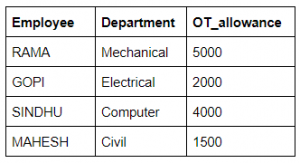What is the output of the following SQL query?
select count(*) from ((select Employee, Department from Overtime_allowance) as S
natural join (select Department, OT_allowance from Overtime_allowance) as T);
 A 16 B 4 C 8 D None of the above
Database-Management-System       SQL
Question 7 Explanation:Common attributes in both the table column are the department. So, we apply natural join, it will give the output as common tuples in both the table S and R.
 Question 8
Which symbol denote derived attributes in the ER Model?
 A Double ellipse B Dashed ellipse C Squared ellipse D An ellipse with attribute name underlined
Database-Management-System       ER-Model
Question 8 Explanation:
Derived attributes are depicted by dashed ellipse.
Example:Question 9
The symmetric difference of sets A = {1, 2, 3, 4, 5, 6, 7, 8} and B = {1, 3, 5, 6, 7, 8, 9} is
 A {1, 3, 5, 6, 7, 8} B {2, 4, 9} C {2, 4} D {1, 2, 3, 4, 5, 6, 7, 8, 9}
Engineering-Mathematics       Sets-And Relation
Question 9 Explanation:
Symmetrical Difference of A and B=(A–B)∪(B–A)Question 10
The problems 3-SAT and 2-SAT are
 A Both NP-complete B Both in P C NP-complete and in P, respectively D Undecidable and NP-complete, respectively
Algorithms       NP complete
Question 10 Explanation:
→ 2-SAT is the first non polynomial problem is solved in polynomial time.
→ 3-SAT problem is NP- complete problem
 Question 11
Given the following statements:
S1 : Every context-sensitive language L is recursive
S2 : There exists a recursive language that is not context-sensitive
Which statements are true?
 A Only S1 is correct B Only S2 is correct C Both S1 and S2 are not correct D Both S1 and S2 are correct
Theory-of-Computation       Languages-and-Grammars
Question 11 Explanation:
According to the Chomsky hierarchy, both the statements are correct.Question 12
Which one of the following is FALSE?
 A There is a unique minimal DFA for every regular language B Every NFA can be converted to an equivalent PDA C The complement of every context-free language is recursive D Every non-deterministic PDA can be converted to an equivalent deterministic PDA
Theory-of-Computation       Equivalence-of-Languages
Question 12 Explanation:
→ NPDA is more powerful than DPDA because
1. DPDA accept only a proper subset of CFL's ie. LL grammars.
2. NPDA can accept any CFL, which makes them more powerful over DPDA
3. Every NPDA can not be converted to an equivalent DPDA
 Question 13
In some programming languages, an identifier is permitted to be a letter followed by any number of letters or digits. If L and D denotes the set of letters and digit respectively. Which of the following expression defines an identifier?
 A (L + D)* B (L.D)* C L(L + D)* D L(L.D)*
Theory-of-Computation       Regular-Expression
Question 13 Explanation:
Option C is correct because it indicates L followed by (L + D)* where * means 0 or more occurences of L or D.
 Question 14
The recurrence relation that arises in relation with the complexity of binary search is:
 A T(n)=2T(n/2)+k , where k is constant B T(n)=T(n/2) +k, where k is constant C T(n)=T(n/2)+logn D T(n)=T(n/2)+n
Algorithms       Searching
Question 14 Explanation:
Binary search in a sorted array
The time to search in an array of ‘n’ elements is equal to the time to search in an array of n/2 elements plus k comparison.
T(n)=T(n/2)+k // k is constant
 Question 15
Which one of the following in-place sorting algorithms needs the minimum number of swaps?
 A Insertion Sort B Quick Sort C Heap Sort D Selection Sort
Algorithms       Sorting
Question 15 Explanation:
Selection sort requires minimum number of swaps i.e O(n)Question 16
Given two statements:
(i) Insertion of an element should be done at the last node in a circular list
(ii) Deletion of an element should be done at the last node of the circular list
 A Both are true B Both are false C First is false and second is true D None of the above
Question 16 Explanation:
There are three situation for inserting element and deleting an element in Circular linked list.
1.Insertion at the front of Circular linked list.
2.Insertion in the middle of the Circular linked list.
3.Insertion at the end of the Circular linked list.
 Question 17
Which of the following data structure is useful in traversing a given graph by breadth-first search?
 A Stack B List C Queue D None of the above
Data-Structures       Graphs
Question 17 Explanation: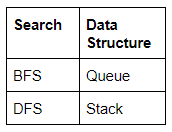Question 18
How many 128×8 bit RAMs are required to design 32K×32 bit RAM?
 A 512 B 1024 C 128 D 32
Digital-Logic-Design       RAM
Question 18 Explanation:
Step-1: RAM size required is 32Kx32 bits
= 32x1024x32 bits. [Note: 1k=1024 bytes]

Step-2: Given RAM chip capacity is 128x8 bits
Step-3: Required size/Given size
=(32*1024*32) / (128*8)
=1024
 Question 19
The most appropriate matching for the following pairs :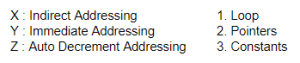A X − 3, Y − 2, Z −1 B X − 2, Y − 3, Z −1 C X − 3, Y −1, Z − 2 D X − 2, Y −1, Z − 3
Question 19 Explanation:
Indirect addressing means that the address of the data is held in an intermediate location so that the address is first 'looked up' and then used to locate the data itself.
Immediate Addressing. An immediate operand has a constant value or an expression. When an instruction with two operands uses immediate addressing, the first operand may be a register or memory location, and the second operand is an immediate constant. Auto increment or decrements: can be one by using loops.
 Question 20
Which interrupt in 8085 Microprocessor is unmaskable?
 A RST 5.5 B RST 7.5 C TRAP D Both (a) and (b)
Computer-Organization       Microprocessor
Question 20 Explanation:
Interrupts are the signals generated by the external devices to request the microprocessor to perform a ask. There are 5 interrupt signals. Given low priority to high is
1. INTR
2. RST 5.5
3. RST 6.5
4. RST 7.5
5. TRAP
Maskable Interrupts: They can be enabled or disabled by software INTR,RST 5.5,RST 6.5 and RST 7.5
 Question 21
A cache memory needs an access time of 30 ns and main memory 150 ns, what is the average access time of CPU (assume hit ratio = 80%)?
 A 60 B 30 C 150 D 70
Computer-Organization       Cache
Question 21 Explanation:
Step-1: Hit ratio 80%=0.8 and Miss ratio=20%=0.2
Access time=30ns
Main memory=150ns
Step-2: CPU access time = (Hit ratio*access time) + (Miss ratio*access time+Main memory)
= (0.8*30) + (0.2*(30+150))
= 60 ns
 Question 22
Which one of the following Boolean expressions is NOT a tautology?
 A ((a → b) ∧ (b → c)) → (a → c) B (a ↔ c) → ( ¬b → (a ∧ c)) C (a ∧ b ∧ c) → (c ∨ a) D a → (b → a)
Engineering-Mathematics       Propositional-Logic
Question 22 Explanation:
Tautology: A universal truth in formal logic.Note: Above (A↔C) →( ¬B→(A∧C)) is not tautology.
 Question 23
What is the minimum number of two-input NAND gates used to perform the function of two input OR gate
 A One B Two C Three D Four
Digital-Logic-Design       Logic-Gates
Question 23 Explanation:Question 24
When two n-bit binary numbers are added the sum will contain at the most
 A n bits B (n+3) bits C (n+2) bits D (n+1) bits
Digital-Logic-Design       Number-Systems
Question 24 Explanation:
→ When two n-bit binary numbers are added the sum will contain at the most (n+1) bits
Example = 2 Decimal numbers are (7)10 and (7)10
= Equivalent binary numbers are (111)2 + (111)2
= Adding two binary numbers, the final result will be n+1 number (1110)2
 Question 25
The 2-input XOR has a high output only when the input values are
 A low B high C same D different
Digital-Logic-Design       Logic-Gates
Question 25 Explanation:
The 2-input XOR(⊕) has a high output only when the input values are different.Question 26
Physical topology of FDDI is?
 A Bus B Ring C Star D None of the above
Computer-Networks       Topologies
Question 26 Explanation:
Fiber Distributed Data Interface
FDDI provides a 100 Mbit/s optical standard for data transmission in local area network that can extend in range up to 200 kilometers (120 mi). Although FDDI logical topology is a ring based token network, it did not use the IEEE 802.5 token ring protocol as its basis; instead, its protocol was derived from the IEEE 802.4 token bus timed token protocol.
In addition to covering large geographical areas, FDDI local area networks can support thousands of users. FDDI offers both a Dual-Attached Station (DAS), counter-rotating token ring topology and a Single-Attached Station (SAS), token bus passing ring topology.
 Question 27
In networking terminology UTP means
 A Ubiquitous Teflon port B Uniformly terminating port C Unshielded twisted pair D Unshielded T-connector port
Computer-Networks
Question 27 Explanation:
Unshielded Twisted Pair(UTP): It consists of two unshielded wires twisted around each other. Due to its low cost, UTP cabling is used extensively for local-area networks (LANs) and telephone connections. UTP cabling does not offer as high bandwidth or as good protection from interference as coaxial or fiber optic cables, but it is less expensive and easier to work.
 Question 28
The default subnet mask for a class B network can be
 A 255.255.255.0 B 255.0.0.0 C 255.255.192.0 D 255.255.0.0
Computer-Networks       Subnetting
Question 28 Explanation:
→ Class A addresses only include IP starting from 1.x.x.x to 126.x.x.x only. The IP range 127.x.x.x is reserved for loopback IP addresses.
→ Class B IP Addresses range from 128.0.x.x to 191.255.x.x. The default subnet mask for Class B is 255.255.x.x.
→ Class C IP addresses range from 192.0.0.x to 223.255.255.x. The default subnet mask for Class C is 255.255.255.x.
 Question 29
If there are n devices (nodes) in a network, what is the number of cable links required for a fully connected mesh and a star topology respectively
 A n(n−1)/ 2, n−1 B n, n−1 C n−1, n D n−1, n(n −1)/2
Computer-Networks       Topologies
Question 29 Explanation:
→ Fully connected mesh we can also called complete graph. So, it has total number of cable links with n nodes are n(n-1)/2
→ In a star topology total number of cable links are (n-1)
 Question 30
Which of the following protocol is used for transferring electronic mail messages from one machine to another?
 A TELNET B FTP C SNMP D SMTP
Computer-Networks       Application-Layer-Protocol
Question 30 Explanation:
TELNET→ Telephone network
FTP→ File transfer protocol
SNMP→ Simple network management protocol
SMTP→ Simple mail transfer protocol Simple Mail Transfer Protocol (SMTP): is an Internet standard for email transmission.
→ Mail servers and other mail transfer agents use SMTP to send and receive mail messages on TCP port 25.
 Question 31
Which media access control protocol is used by IEEE 802.11 wireless LAN?
 A CDMA B CSMA/CA C ALOHA D None of the above
Computer-Networks       Wi-Fi
Question 31 Explanation:
This layer provides the same function as the MAC layer in the wired LAN environment. IEEE 802.11, instead of using Ethernet's CSMA/CD, specifies a scheme called CSMA/CA.
CA stands for Collision Avoidance. (In the wireless environment, since a station may not be able to hear all other stations, CD, Collision Detection is not feasible)
 Question 32
An Ethernet frame that is less than the IEEE 802.3 minimum length of 64 octets is called
 A Short frame B Small frame C Mini frame D Runt frame
Computer-Networks       Ethernet
Question 32 Explanation:
A runt frame is an Ethernet frame that is less than the IEEE 802.3 minimum length of 64 octets. Runt frames are most commonly caused by collisions; other possible causes are a malfunctioning network card, buffer underrun, duplex mismatch or software issues.
 Question 33
Match with the suitable one:A A-2, B-3, C-4, D-1 B A-2, B-4, C-3, D-1 C A-3, B-4, C-1, D-2 D A-3, B-1, C-4, D-2
Computer-Networks       Routing
Question 33 Explanation:
Internet Group Management Protocol (IGMP) is a communications protocol used by hosts and adjacent routers on IPv4 networks to establish multicast group memberships. IGMP is an integral part of IP multicast.
Open Shortest Path First (OSPF) is a routing protocol for Internet Protocol (IP) networks. It uses a link state routing (LSR) algorithm and falls into the group of interior gateway protocols (IGPs), operating within a single autonomous system (AS).
Border Gateway Protocol (BGP) is a standardized exterior gateway protocol designed to exchange routing and reachability information among autonomous systems (AS) on the Internet.
Routing Information Protocol (RIP) is one of the oldest distance vector routing protocols which employ the hop count as a routing metric. RIP implements the split horizon, route poisoning and hold down mechanisms to prevent incorrect routing information from being propagated.
 Question 34
MD5 is a widely used hash function for producing hash value of
 A 64 bits B 128 bits C 512 bits D 1024 bits
Computer-Networks       Network-Security
Question 34 Explanation:
→ The Message Digest(MD5) algorithm is a widely used hash function producing a 128-bit hash value. Although MD5 was initially designed to be used as a cryptographic hash function, it has been found to suffer from extensive vulnerabilities.
→ It can still be used as a checksum to verify data integrity, but only against unintentional corruption. It remains suitable for other non-cryptographic purposes, for example for determining the partition for a particular key in a partitioned database.
 Question 35
Which protocol suite designed by IETF to provide security for a packet at the Internet layer?
 A IPsec B NetSec C PacketSec D SSL
Question 35 Explanation:
→ The Internet Engineering Task Force(IETF) IP Security Working Group formed to standardize these efforts as an open, freely available set of security extensions called IPsec.
 Question 36
Pretty Good Privacy (PGP) is used in
 A Browser security B FTP security C Email security D None of the above
Computer-Networks
Question 36 Explanation:
→ Pretty Good Privacy (PGP) is an encryption program that provides cryptographic privacy and authentication for data communication. PGP is used for signing, encrypting, and decrypting texts, e-mails, files, directories, and whole disk partitions and to increase the security of e-mail communications. Phil Zimmermann developed PGP in 1991.
→ It uses Digital Signatures to provide authentication and integrity checking basically to check if the message is actually sent by the person or entity claimed to be the sender.
 Question 37
What is WPA?
 A wired protected access B wi-fi protected access C wired process access D wi-fi process access
Computer-Networks       Wi-Fi
Question 37 Explanation:
→ WPA stands for Wi-Fi protected access. It is a security standard for computing devices equipped with wireless internet connection.
→ WPA provides data encryption and user authentication to the computing devices connected to a wi-fi network.
→ WPA also includes a Message Integrity Check, which is designed to prevent an attacker from altering and resending data packets. This replaces the cyclic redundancy check (CRC) that was used by the WEP standard.
 Question 38
Estimation of software development effort for organic software in basic COCOMO is
 A E = 2.0(KLOC)1.05 Per Month B E = 3.4(KLOC)1.06 Per Month C E = 2.4(KLOC)1.05 Per Month D E = 2.4(KLOC)1.07 Per Month
Software-Engineering       COCOMO-Model
Question 38 Explanation:
The basic COCOMO equations take the form
1. Effort Applied (E) = ab(KLOC)bb [ man-months ]
2. Development Time (D) = cb(Effort Applied)db [months]
3. People required (P) = Effort Applied / Development Time [count]

where, KLOC is the estimated number of delivered lines (expressed in thousands ) of code for project. The constants ab, bb, cb and db are given in the following table (note: the values listed below are from the original analysis, with a modern reanalysis producing different values)Question 39
XPath is used to navigate through elements and attributes in
 A XSL document B XML document C XHTML document D XQuery document
Web-Technologies       XML
Question 39 Explanation:
XPath
1. XPath stands for XML Path Language
2. XPath uses "path like" syntax to identify and navigate nodes in an XML document
3. XPath contains over 200 built-in functions
4. XPath is a major element in the XSLT standardQuestion 40
What is the output of this C++ program?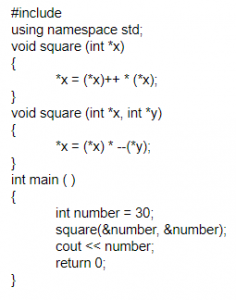A 910 B 920 C 870 D 900
Programming-for-Output-Problems       Functions
Question 40 Explanation:
Step-1: In main function, number variable has been initialized to 30.
Step-2: We pass address of number as parameters in the square function. As per the concept of function overloading in C++ the second square function will be executed.
Step-3: --(*y) will be executed first since decrement operator has the higher precedence than multiplication.
*x = (*x) * --(*y);
*x = 30 * 29;
*x = 870
Note: x is a pointer variable and it holds the address of the variable number.
 Question 41
Which of the following operator(s) cannot be overloaded?
 A . (Member Access or Dot operator) B ?: (Ternary or Conditional Operator ) C :: (Scope Resolution Operator) D All of the above
OOPS       Properties
Question 41 Explanation:
. (Member Access or Dot operator), ?: (Ternary or Conditional Operator ) and :: (Scope Resolution Operator) are ternary operators. It can’t be overloaded.
 Question 42
Which of the following UML 2.0 diagrams capture behavioural aspects of a system?
 A Use Case Diagram, Object Diagram, Activity Diagram, and State Machine Diagram B Use Case Diagram, Activity Diagram, and State Machine Diagram C Object Diagram, Communication Diagram, Timing Diagram, and Interaction diagram D Object Diagram, Composite Structure Diagram, Package Diagram, and Deployment Diagram
Software-Engineering       Software-design
Question 42 Explanation:
UML diagram are two types:Question 43
Which of the following is associated with objects?
 A State B Behaviour C Identity D All of the above
OOPS       Class-and-object
Question 43 Explanation:
→ An object can be a variable, a data structure, a function, or a method, and as such, is a value in memory referenced by an identifier.
→ In the class based object-oriented programming paradigm, object refers to a particular instance of a class, where the object can be a combination of variables, functions, and data structures.
→ An object has state, exhibits some well defined behavior, and has a unique identity.
 Question 44
Which one of these is characteristic of RAID 5?
 A Dedicated Parity B Double Parity C Hamming code Parity D Distributed Parity
Computer-Organization       RAID
Question 44 Explanation:
RAID 0: Disk Striping or non-redundant striping
RAID 1: Disk Mirroring
RAID 2: Memory style error correcting codes
RAID 3: Bit interleaved parity
RAID 4: Block interleaved parity
RAID 5: Block interleaved distributed parity
RAID 6: P+Q redundancy
 Question 45
SATA is the abbreviation of
Computer-Networks
Question 45 Explanation:
→ Serial ATA (Serial Advanced Technology Attachment or SATA) is a standard for connecting and transferring data from hard disk drives (HDDs) to computer systems.
→ As its name implies, SATA is based on serial signaling technology, unlike Integrated Drive Electronics (IDE) hard drives that use parallel signaling.
 Question 46
Capability Maturity Model (CMM) is a methodology to
 A develop and refine an organization’s software development process B develop the software C test the software D All of the above
Software-Engineering       Software-design
Question 46 Explanation:
CMM stands for Capability Maturity Model is a process model which specifies the process improvement approach in software development.
CMM levels:
1. Initial
2. Repeatable
3. Defined
4. Managed
5. Optimizing
 Question 47
What problem is solved by Dijkstra banker’s algorithm?
 A Cache coherence B Mutual exclusion C Deadlock recovery D Deadlock avoidance
Question 47 Explanation:
Deadlock avoidance is solved by Dijkstra banker’s algorithm
 Question 48
The number of swappings needed to sort the numbers 8, 22, 7, 9, 31, 5, 13 in ascending order, using bubble sort is
 A 11 B 12 C 13 D 10
Algorithms       Sorting
Question 48 Explanation:
Step-1: 8,7,22,9,31,5,13
Step-2: 8,7,9,22,31,5,13
Step-3: 8,7,9,22,5,31,13
Step-4: 8,7,9,22,5,13,31
Step-5: 7,8,9,22,5,13,31
Step-6: 7,8,9,5,22,13,31
Step-7: 7,8,9,5,13,22,31
Step-8: 7,8,5,9,13,22,31
Step-9: 7,5,8,9,13,22,31
Step-10: 5,7,8,9,12,22,31
Note:Total 10 swaps are required to sort the array.
 Question 49
Consider the following treeIf the post order traversal gives ab-cd*+ then the label of the nodes 1,2,3,… will be
 A +,-,*,a,b,c,d B a,-,b,+,c,*,d C a,b,c,d,-,*,+ D -,a,b,+,*,c,d
Data-Structures        Binary-Trees
Question 49 Explanation:
Step-1: Post order traversals yields from left,right and root.Step-2: The post order sequence is 4526731. The same we have to take it for above constraint.
Step-3: Then the sequence will be ab–cd*+ because 1= +, 2= -, 3= *, 4= a, 5=b, 6=c and 7=d.
 Question 50
What is the output of the following program?A 10 and 11 B 10 C 11 D 11 and 11
Operating-Systems       System-Calls
Question 50 Explanation:
→ The purpose of the fork system call is to create a child process.
→ The parent process fork call will return process ID which will make if condition false then parent process will print 10
→ The child process will execute the next instruction is a++ because in the child process if the condition is not tested. Execution starts from next instruction and it will print 11
 Question 51
Given the reference to the following pages by a program 0, 9, 0, 1, 8, 1, 8, 7, 8, 7, 1, 2, 8, 2, 7, 8, 2, 3, 8, 3 How many page faults will occur if the program has three-page frames available to it and uses an optimal replacement?
 A 7 B 8 C 9 D None of these
Operating-Systems       Page-Replacement-algorithm
Question 51 Explanation:
Optimal page replacement: Replace the page that will not be used for the longest period of time.Note: Total 7-page faults will occur.
 Question 52
Consider the following C functionA Call swap (a, b) B Call swap (&a, &b) C swap(a, b) cannot be used as it does not return any value D swap(a, b) cannot be used as the parameters passed by value
Programming       Functions
Question 52 Explanation: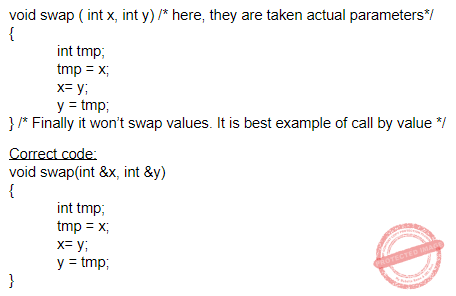Question 53
What does the following C-statement declare? int (*f) (int*);
 A A function that takes an integer pointer as an argument and returns an integer B A function that takes an integer as argument and returns an integer pointer C A pointer to a function that takes an integer pointer as an argument and returns an integer D A function that takes an integer pointer as an argument and returns a function pointer
Programming       Declaration
Question 53 Explanation:
→ int (*f) (int*); f is a pointer to a function that takes an integer pointer as an argument and returns an integer
→ int (*p)(char *a); p ia a pointer to a function that takes an argument as a pointer to a character and returns an integer.
 Question 54
Mutual exclusion problem occurs
 A Between two disjoint processes that do not interact B Among processes that share resources C Among processes that do not use the same resource D Between two processes that uses different resources of different machine
Operating-Systems       Process-Synchronization
Question 54 Explanation:
→ Mutual exclusion is used to avoid the concurrent use of the same resources. But sometimes the problem occurred in mutual exclusion when process or program is not sharing the same resources.
 Question 55
(1217)8 is equivalent to
 A (1217)16 B (028F)16 C (2297)1o D (0B17)16
Digital-Logic-Design       Number-Systems
Question 55 Explanation:
(1217)8=(001 010 001 111)2
=(0010 1000 1111)2
=(2 8 F)16
 Question 56
Which of the following is not a life cycle model?
 A Spiral model B Prototyping model C Waterfall model D Capability maturity model
Software-Engineering       Software-process-models
Question 56 Explanation:
→ SDLC is a process followed for a software project, within a software organization. It consists of a detailed plan describing how to develop, maintain, replace and alter or enhance specific software. The life cycle defines a methodology for improving the quality of software and the overall development process.
1. Waterfall model
2. Iterative model
3. Spiral model
4. Incremental model
5. Modified waterfall model
7. Agile model
→ CMM stands for Capability Maturity Model is a process model which specifies the process improvement approach in software development.
 Question 57
The best data structure to check whether an arithmetic expression has balanced parenthesis is a
 A Queue B Stack C Tree D List
Data-Structures       Queues-and-Stacks
Question 57 Explanation:
→ The stack is the best data structure to validate the arithmetic expression.
→ While evaluating when left parentheses occur then it pushes into the stack when right parentheses occur pop from the stack. While at the end there is empty in the stack.
 Question 58
The cyclomatic complexity of each of the modules X and Y shown below is 10. What is the cyclomatic complexity of the sequential integration shown on the right-hand side?A 21 B 19 C 20 D 10
Software-Engineering       Software-quality
Question 58 Explanation:
Cyclomatic complexity uses 3 formulas
1. Number of regions + 1
2. Predicate + 1
3. Edges-Vertices+2

But here they already given complexity. So each module complexity is 10.
There are 2 modules named A and B having decision points are (10-1)+(10-1).
Cyclomatic complexity of the sequential integration is = Number of decision points + 1
= 18+ 1
= 19
 Question 59
In software maintenance tackling the changes in the hardware or software environment where the software works, is
 A Corrective maintenance B Perfective maintenance C Adaptive maintenance D Preventive maintenance
Software-Engineering       Software-configuration-management
Question 59 Explanation:
Software Maintenance activities into four classes:
1. Adaptive – modifying the system to cope with changes in the software environment
2. Perfective – implementing new or changed user requirements which concern functional enhancements to the software
3. Corrective – diagnosing and fixing errors, possibly ones found by users
4. Preventive – increasing software maintainability or reliability to prevent problems in the future
 Question 60
What will be the output of the following C code?A 10 11 12 13 14 B 10 10 10 10 10 C 0 1 2 3 4 D Compilation error
Programming       Control Flow
Question 60 Explanation:
Step-1: We are initialized i=0 in for loop. It means condition true because it is less than 5.
Step-2: Inside the for loop we are assigning again I value is 10 and printing value i.
Iteration-1: We are printing value 10 then increment by 1
Iteration-2: We are clearing previous value and assigning value 10. Printing value is 10;
Iteration-5: We are clearing previous value and assigning value 10. Printing value is 10
Step-3: output is 1010101010
 Question 61
What does the following program do when the input is unsigned 16-bit integer?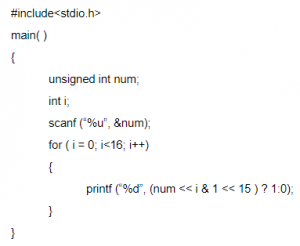A It prints all even bits from num B It prints all odd bits from num C It prints binary equivalent of num D None of the above
Programming       Control Flow
Question 61 Explanation:
Step-1: Take n value initially 14(any number we can take but we are taken 14)
Step-2: 14<16
Step-3: It will print 00000000 00001110
 Question 62
What is the output of the following program?A 20 10 10 B 20 10 20 C 20 20 20 D 10 10 10
Programming       Functions
Question 62 Explanation:
Step-1: Every program starts execution from main() function.
Step-2: By default tmp value 20 will print initially.
Step-3: It calls fun() then it will print 10
Step-4: Again we are in main() function and printing value 20. Actually, the static value will return because its scope is a lifetime. But we are given tmp value as global. So, it prints 20 instead of 10.
 Question 63
Which product metric gives the measure of the average length of words and sentence in documents?
 A SCI number B Cyclomatic complexity C LOC D Fog index
Question 63 Explanation:
→ In linguistics, the Gunning fog index is a readability test for English writing. The index estimates the years of formal education a person needs to understand the text on the first reading
→ FOG index gives the average length of words and sentences in a document. The Fog Index is a readability test which predicts whether a document is easy to read or if it is difficult to read.
 Question 64
Consider a disk system with 100 cylinders. The request to access the cylinders occur in the following sequences 4, 34, 10, 7, 19, 73, 2, 15, 6, 20 Assuming the head is currently at cylinder 50, what is the time taken to satisfy all requests if it takes 1 ms to move from one cylinder to adjacent one and shortest seek time first algorithm is used.
 A 95 msec B 119 msec C 233 msec D 276 msec
Computer-Organization       Disk-Scheduling
Question 64 Explanation:
The given sequence is
4, 34, 10,7, 19, 73, 2, 15, 6, 20
Arrange the sequence in order
2, 4, 6, 10, 15, 19, 20, 34, 73⇒ 1 ms to move from one cylinder to adjacent one
⇒ (16*1)+(14*1)+(1*1)+(4*1)+(5*1)+(3*1)+(1*1)+(2*1)+(2*1)+(71*1)
⇒ 16+14+1+4+5+3+1+2+2+71
⇒ 119 ms
 Question 65
A B-Tree used as an index for a large database table has four levels including the root node. If a new key is inserted in this index, then the maximum number of nodes that could be newly created in the process are
 A 5 B 4 C 1 D 2
Database-Management-System       B-and-B+-Trees
Question 65 Explanation:
No. of nodes in children of a node = no. of keys in parent node + 1
Here, the tree has 4 levels, then 4+1=5 nodes to be present in the newly created process.
 Question 66
A critical region
 A is a piece of code which only one process executes at a time B is a region prone to deadlock C is a piece of code which only a finite number of processes execute D is found only in Windows NT operating system
Operating-Systems       Process-Synchronization
Question 66 Explanation:
A critical region is a piece of code which only one process executes at a time. It cannot be executed by more than one process at a time.
 Question 67
Choose the equivalent prefix form of the following expression (a + (b − c))* ((d − e)/(f + g − h))
 A * +a − bc /− de − +fgh B * +a −bc − /de − +fgh C * +a − bc /− ed + −fgh D * +ab − c /− ed + −fgh
Data-Structures       Prefix-Postfix-Expression
Question 67 Explanation:
→ An expression is called the prefix expression if the operator appears in the expression before the operands.Question 68
We use malloc and calloc for
 A Dynamic memory allocation B Static memory allocation C Both dynamic and static memory allocation D None of the above
Programming       Calloc-Malloc
Question 68 Explanation:
C -Dynamic memory allocation refers to performing manual memory management for dynamic memory allocation in the C programming language via a group of functions in the C standard library, namely
1. Malloc
2. Realloc
3. Calloc
4. Free.
 Question 69
The Linux command “mknod myfifo b 4 16”
 A Will create a character device if the user is root B Will create a named pipe FIFO if the user is root C Will create a block device if the user is root D None of the above
Operating-Systems       Linux-Operating-systems
Question 69 Explanation:
The mknod command makes a directory entry and corresponding inode for a special file. The first parameter is the name of the Name entry device. Select a name that is descriptive of the device. The mknod command has two forms that have different flags.
Syntax: mknod Name { b | c } Major Minor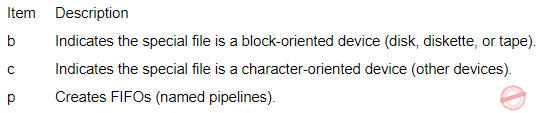Question 70
Which of the following statement is true?
 A Hard real-time OS has less jitter than soft real-time OS B Hard real-time OS has more jitter than soft real-time OS C Hard real-time OS has equal jitter as a soft real-time OS D None of the above
Operating-Systems       Real-Time-Operating-System
Question 70 Explanation:
→ A key characteristic of an RTOS is the level of its consistency concerning the amount of time it takes to accept and complete an application's task; the variability is jitter.
→ A hard real-time operating system has less jitter than a soft real-time operating system. The chief design goal is not high throughput, but rather a guarantee of a soft or hard performance category.
→ An RTOS that can usually or generally meet a deadline is a soft real-time OS, but if it can meet a deadline deterministically it is a hard real-time OS.
 Question 71
Which of these is a super class of all errors and exceptions in the Java language?
 A Runtime Exceptions B Throwable C Catchable D None of the above
OOPS       JAVA
Question 71 Explanation:
The Throwable class is the superclass of all errors and exceptions in the Java language.
 Question 72
Choose the most appropriate HTML tag in the following to create a numbered lists.
 A < dl > B < ul > C < li > D < ol >
Web-Technologies       HTML
Question 72 Explanation:
→ The
tag defines an ordered list. An ordered list can be numerical or alphabetical.
→ The
tag defines an unordered (bulleted) list. Use the
tag together with the
• tag to create unordered lists.
 Question 73
Which of the following algorithm solves the all-pairs shortest path problem?
 A Prim’s algorithm B Dijkstra's algorithm C Bellman-Ford’s algorithm D Floyd-Warshall’s algorithm
Algorithms       Dynamic-Programming
Question 73 Explanation:
Prim's → Minimum Spanning tree
Dijkstra's algorithm → Single source shortest path for only positive values
Bellman Ford’s algorithm → Single source shortest path for either positive values or negative values but not negative weight cycle.
Floyd-Warshall’s algorithm→ All pair shortest path problem
 Question 74
If L and P are two recursively enumerable languages, then they are not closed under
 A Kleene Star L * of L B Intersection L ∩ P C Union L ∪ P D Set Difference
Theory-of-Computation       Recursive-and-Recursively-Enumerable-Language
Question 74 Explanation:
→ Recursively Enumerable problems are not closed under Complementation.
→ Set Difference A – B can be written as A ∩ B’ where B’ denotes complementation of B. So we can say that Recursively Enumerable problems are not closed under Set Difference
 Question 75
In the context of modular software design, which one of the following combinations is desirable?
 A High cohesion and high coupling B High cohesion and low coupling C Low cohesion and high coupling D Low cohesion and low coupling
Software-Engineering       Software-design
Question 75 Explanation:
→ Cohesion is a measure of internal strength within a module, whereas coupling is a measure of inter dependency among the modules.
→ So in the context of modular software design, there should be high cohesion and low coupling.
 Question 76
The output of a lexical analyzer is
 A A parse tree B Intermediate code C Machine code D A stream of tokens
Compiler-Design       Compilers
Question 76 Explanation:
Explanation: The output of a lexical analyzer is a stream of tokens.
 Question 77
The time complexity of computing the transitive closure of binary relation on a set of n elements is known to be
 A O(nlogn) B O(n3/2) C O(n3) D O(n)
Algorithms       Time-Complexity
Question 77 Explanation:
Transitive closure generally uses Floyd-Warshall Algorithm which gives a time complexity of O(n3)
 Question 78
At a particular time, the value of a counting semaphore is 10, it will become 7 after: (a) 3 V operations (b) 3 P operations (c) 5 V operations and 2 P operations (d) 2 V operations and 5 P operations Which of the following option is correct?
 A Only (b) B Only(d) C Both (b) and (d) D None of these
Operating-Systems       Process-Synchronization
Question 78 Explanation:
P: Wait operation decrements the value of the counting semaphore by 1.
V: Signal operation increments the value of counting semaphore by 1.

Current value of the counting semaphore = 10
a) after 3 P operations, value of semaphore = 10-3 = 7
d) after 2 v operations, and 5 operations value of semaphore = 10 + 2 – 5 = 7
 Question 79
In a doubly linked list, the number of pointers affected for an insertion operation will be
 A 4 B 0 C 1 D None of these